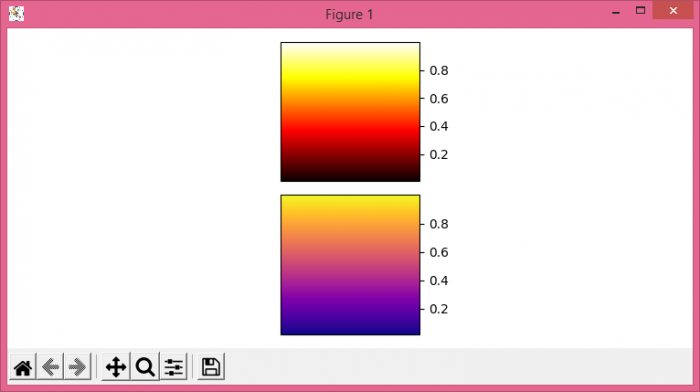# How to show multiple colorbars in Matplotlib?

To show multiple colorbars in matplotlib, we can take the following steps−

• Set the figure size and adjust the padding between and around the subplots.
• Create a figure and a set of subplots.
• Initialize a variable N for the number of sample data.
• Create random data1 using numpy.
• Display data as an image, i.e., on a 2D regular raster, with data1.
• Add a colorbar to a plot.
• Repeat steps 4, 5, and 6, with different datasets and axes.
• To display the figure, use show() method.

## Example

from matplotlib import pyplot as plt
import numpy as np
plt.rcParams["figure.figsize"] = [7.50, 3.50]
plt.rcParams["figure.autolayout"] = True
fig, (ax1, ax2) = plt.subplots(nrows=2, ncols=1)
N = 10
data1 = np.random.rand(N, N)
im1 = ax1.imshow(data1, cmap='hot')
plt.colorbar(im1, cax=ax1)
data2 = np.random.rand(N, N)
im2 = ax2.imshow(data2, cmap='plasma')
plt.colorbar(im2, cax=ax2)
plt.show()

## Output